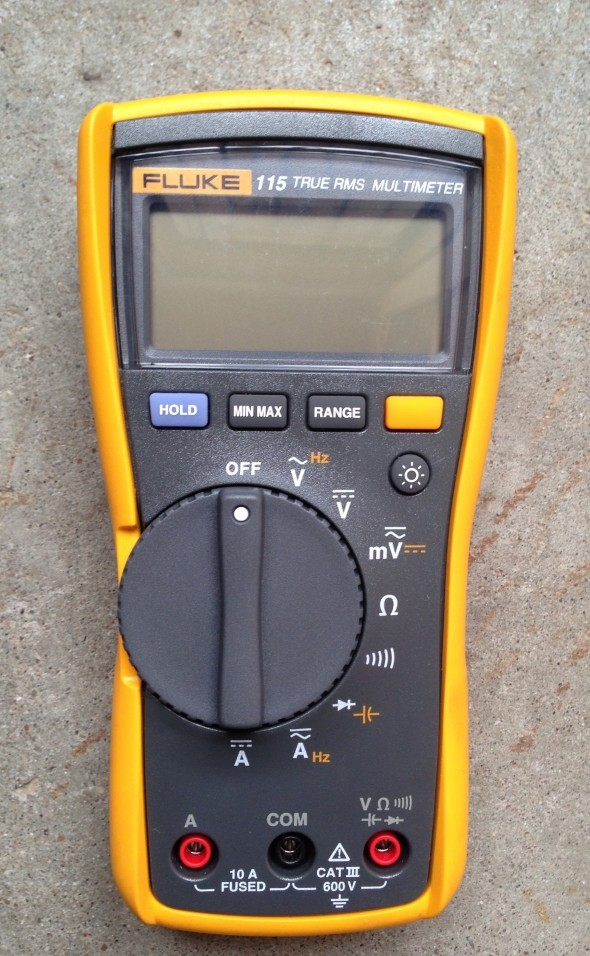# What is millivolts?

A millivolt is a thousandth of a volt. Volts (V) is the measure or the unit of voltage. Voltage is the pressure that pushes current or electrons down a circuit (it is also known as electro motive force – EMF). There are two formulas used to calculate voltage, these are:

Voltage is equal to Power divided by Current and Voltage is equal to Current multiplied by Resistance.

1) V = P / I

2) V= I × RThe image above is a formula triangle that can be used to work out voltage.

### What tool is needed to measure millivolts?

In order to measure millivolts, you will first need a tool that is able to measure millivolts. One of the most common tools used for measuring millivolts is a multimeter. A multimeter is a meter that is able to measure a variety of different things like resistance, current and many other things as well as millivolts.

Multimeters combine a variety of measuring equipment such as a volt meter, Amp meter, ohm meter and other meters all in to one multi-measuring device. This saves you from having to switch between meters every time you need to measure a new unit of measurement, thus, saving time and It also saves space as you will only have to carry one device.

### How can you measure millivolts?

Measuring millivolts is not very difficult and is exactly the same as measuring volts. The first thing you will need to do is get yourself a multimeter. The way you measure millivolts will vary depending on the type of multimeter you use. I recommend using an auto-ranging multimeter because it is the simplest and quickest to use. The second thing you need is a pair of probes, one black and one red. On an auto-ranging multimeter the first thing you have to do is turn the dial (which is generally in the middle of multimeter) to the millivolts symbol which is a small m and a capital V next to each other. After this you need to make sure you have inserted the red and black test probes in their correct sockets. Now that you have done this, you can take a measurement from any extra low voltage circuit or supply and the reading that will be displayed on the multimeter will be in millivolts.

### Why you should use the auto-ranging meter?

In the previous paragraph I discussed how we can measure millivolts. The tool that you need in order to measure millivolts is called a multimeter. There are many types of multimeters that can be used however I only spoke about how to use an auto ranging digital multimeter. The reason for this is that auto-ranging meters are the easiest to use compared to the other types of multimeters.

One of the other types of multimeters are called an analog multimeter and a digital manual ranging multimeter.

An analog multimeter uses a needle that moves along a number line in order to get a measurement. Whatever number it stops on will be the reading for the thing you have measured. The problem with this is that there are too many unit symbols and various number lines which can cause confusion and requires full attention at all times. Additionally, when you use these meters you have to select an appropriate range for the thing you are measuring. For example if I was using a multimeter an expecting to get a reading of 100 volts and the range I selected was set to 10, the needle will move off the scale which means over limit or maximum value. In order for you to get the right reading you have to select the appropriate range, which in this case, would be 250 volts.

The other meter is a digital manual ranging multimeter. This multimeter is slightly better than the analog meter because it uses a digital number screen instead of a needle and a number line. However, this meter requires you to manually select the unit ranges for your readings like the analog meter.

The auto ranging meter has a function that automatically changes the unit range to a range which is suitable for your reading. This means that you don’t have to worry about finding an appropriate range for your reading and instead you can just focus on taking the measurement which will save you a lot of time. In addition to this, this multimeter is very accurate because it uses a digital number display just like the manual ranging multimeter. When you consider these points, anyone would choose the auto-ranging multimeter over the other two multimeters.Image – J.C. Fields

### Why are millivolts needed?

Millivolts and volts are both the same thing however one is used with larger readings (mains powered electrical installations) and the other is used with smaller readings (electronic equipment and their circuits) . The reason why millivolts is needed is that in electricity there will be many times when you will have to read very tiny values which can be very hard to read with standard voltage detectors. When you set the multimeter to millivolts, the reading will be displayed in away that is easy to read for measurements that are very small. But If we did not have millivolts then the readings would have to be displayed in volts. This would be very difficult for a person using a multimeter (or voltage detector) to understand a small measurement because they will have to read a measurement with many zeros. Using millivolts will present the measurement in a simplified form which can be quickly and easily written.